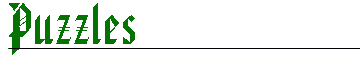[ BACK ]Q1. Flipover      Diagram One is that of the numbers 1,2,3, and 4; each of which is surrounded by eight circles, all of which contain a different letter. The eight circles around each of the four numbers can be 'flipped over' the number itself either vertically or horizontally. For example, in Diagram One, if the letters around the number 1 were flipped vertically, A and F would swap places, B and G would swap places, C and H would swap places and D and E would remain in the same position.   Diagram Two is that of Diagram One after each of the four numbers have been flipped over either vertically or horizontally. In which direction have the letters around each number been flipped over to arrive at the positions shown is diagram Two?Q2. Laser Maze?       The diagram below represents an aerial view of a room which has been divided into 100 squares, as shown by the dotted lines. In each of the squares there should be a dot marked on the floor in the centre of the square, or a double-sided mirror. When all of the dots and mirrors are in their correct places, a laser beam can enter the room at square J10. At the same time, the laser beam shines over each and every dot marked on the floor. When the beam reaches a mirror it 'bounces off' at an angle of 90 degrees, but it never crosses itself. If the beam hits the outside wall it is absorbed by the wall and can go no further.   Fourteen of the dots/mirrors are not in place: five dots (X), six mirrors from lower left to upper right (Y), and three mirrors from upper left to lower right (Z). See if you can determine which of the squares containing '?' should be replaced by:   1. X squares  2. Y squares  3. Z squares     Q3. Stationary Stationery   The other day, the stationery clerk asked George, Fred and Arthur if they would carry some parcels of stationery to the typists' office. There were six parcels in all: one large parcel, which weighed the same as the two medium-sized parcels, which in turn weighed the same as the three small-sized parcels. The stationery clerk then said that one person could carry the large parcel, one person could carry the two medium-sized parcels and one person could carry the three small parcels; that way all three would be carrying the same weight of stationery anyway and said that they would only carry the stationery to the typists on the following conditions-  George would only carry the two medium-sized parcels if Fred carried the three small parcels. Fred would only carry the three small parcels if George carried the large parcel. George would only carry the large parcel if Arthur would carry the two medium-sized parcels. Arthur would carry the two medium-sized parcel if Fred carried the large parcel. George would only carry the three small parcels if Arthur carried the large parcel. All this time the stationery remained stationary. Eventually the stationery clerk came up with a solution to keep all three happy. Who carried what to the typists?      Q4. Letter Boxes  When the diagram below is complete, each column and row should contain the letters A, B, C, D, E, F, G, H, I and J. See if you can complete the diagram by fitting the nine blocks of nine letters into the nine highlighted squares, then filling in the spaces along the top row and in the first column.Q5. Logic Box  Using the following clues, place the letters A to I inclusive into the grid. G is above I and to the right of B. C is to the right of H and above I which is to the left of D. B is to the left of E. H is above F and B. A is above E. ('Above' refers to two letters in the same column. 'Left of/right of' refers to two letters in the same row.)[ Homepage ][ Kids Section]
[ Teachers Section ][ Resources ]